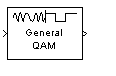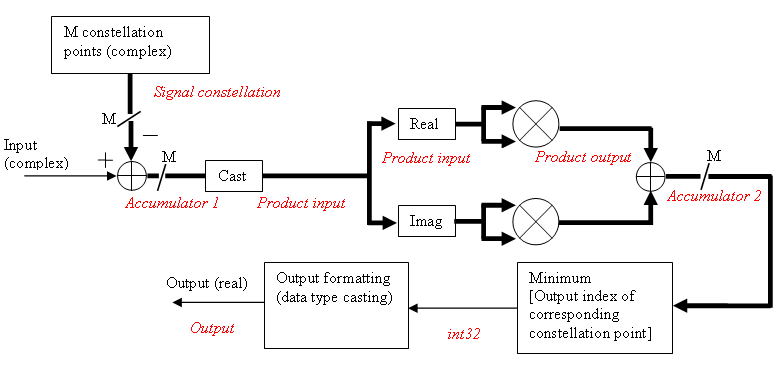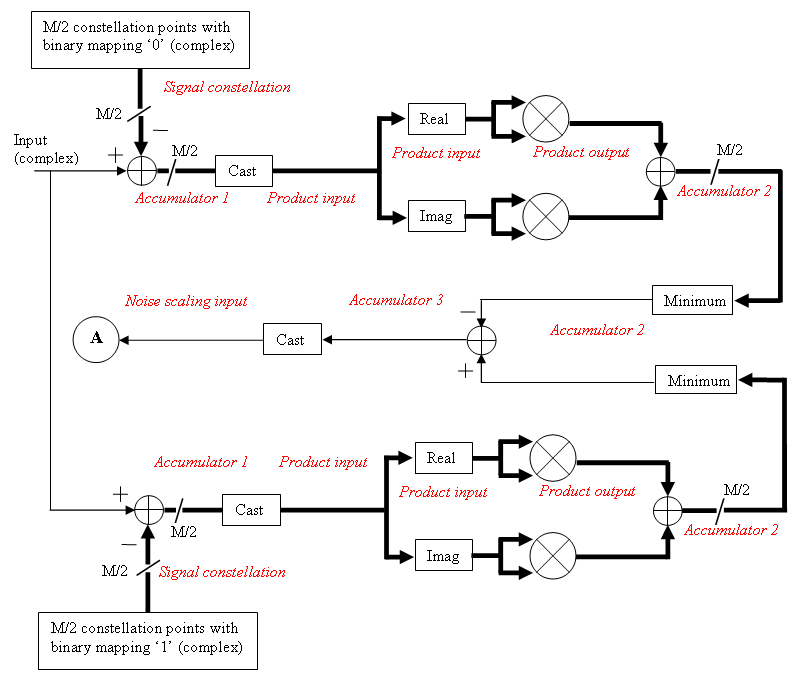General QAM Demodulator Baseband

Demodulate QAM-modulated data

Library

AM, in Digital Baseband sublibrary of Modulation

•Description

The General QAM Demodulator Baseband block demodulates a signal that was modulated using quadrature amplitude modulation. The input is a baseband representation of the modulated signal.

The input must be a discrete-time complex signal. The Signal constellation parameter defines the constellation by listing its points in a length-M vector of complex numbers. The block maps the mth point in the Signal constellation vector to the integer m-1.

This block accepts a scalar or column vector input signal. For information about the data types each block port supports, see the Supported Data Types table on this page.

Parameters

Signal constellation

A real or complex vector that lists the constellation points.

Output type

Determines whether the block produces integers or binary representations of integers.

If you set this parameter to Integer, the block produces integers.

If you set this parameter to Bit, the block produces a group of K bits, called a binary word, for each symbol, when Decision type is set to Hard decision. If Decision type is set to Log-likelihood ratio or Approximate log-likelihood ratio, the block outputs bitwise LLR and approximate LLR, respectively.

Decision type

This field appears when Bit is selected in the pull-down list Output type.

Specifies the use of hard decision, LLR, or approximate LLR during demodulation. See Exact LLR Algorithm and Approximate LLR Algorithm in the Communications Toolbox User's Guide for algorithm details.

Noise variance source

This field appears when you set Approximate log-likelihood ratio or Log-likelihood ratio for Decision type.

When you set this parameter to Dialog, you can then specify the noise variance in the Noise variance field. When you set this option to Port, a port appears on the block through which the noise variance can be input.

Noise variance

This parameter appears when the Noise variance source is set to Dialog and specifies the noise variance in the input signal. This parameter is tunable in normal mode, Accelerator mode and Rapid Accelerator mode.

If you use the Simulink® Coder™ rapid simulation (RSIM) target to build an RSIM executable, then you can tune the parameter without recompiling the model. This is useful for Monte Carlo simulations in which you run the simulation multiple times (perhaps on multiple computers) with different amounts of noise.

The LLR algorithm involves computing exponentials of very large or very small numbers using finite precision arithmetic and would yield:

• Inf to -Inf if Noise variance is very high

• NaN if Noise variance and signal power are both very small

In such cases, use approximate LLR, as its algorithm does not involve computing exponentials.

Fixed-Point Signal Flow Diagrams

Fixed-Point Signal Flow Diagram for Hard Decision ModeNote

In the figure above, M represents the size of the Signal constellation .

The general QAM Demodulator Baseband block supports fixed-point operations for computing Hard Decision (Output type set to Bit and Decision type is set to Hard decision) and Approximate LLR (Output type is set to Bit and Decision type is set to Approximate Log-Likelihood ratio) output values. The input values must have fixed-point data type for fixed-point operations.

Note

Fixed-Point operations are NOT yet supported for Exact LLR output values.

Fixed-Point Signal Flow Diagram for Approximate LLR ModeNote

In the figure above, M represents the size of the Signal constellation.

Fixed-Point Signal Flow Diagram for Approximate LLR Mode: Noise Variance Operation ModesNote

If Noise variance is set to Dialog, the block performs the operations shown inside the dotted line once during initialization. The block also performs these operations if the Noise variance value changes during simulation.

Data Types Attributes

Output

The block supports the following Output options:

When you set the parameter to Inherit via internal rule (default setting), the block inherits the output data type from the input port. The output data type is the same as the input data type if the input is of type single or double.

For integer outputs, you can set this block's output to Inherit via internal rule (default setting), Smallest unsigned integer, int8, uint8, int16, uint16, int32, uint32, single, and double.

For bit outputs, when you set Decision type to Hard decision, you can set the output to Inherit via internal rule, Smallest unsigned integer, int8, uint8, int16, uint16, int32, uint32, boolean, single, or double.

When you set Decision type to Hard decision or Approximate log-likelihood ratio and the input is a floating point data type, then the output inherits its data type from the input. For example, if the input is of data type double, the output is also of data type double. When you set Decision type to Hard decision or Approximate log-likelihood ratio, and the input is a fixed-point signal, the Output parameter, located in the Fixed-Point algorithm parameters region of the Data-Type tab, specifies the output data type.

When you set the parameter to Smallest unsigned integer, the output data type is selected based on the settings used in the Hardware Implementation pane of the Configuration Parameters dialog box. If you select ASIC/FPGA in the Hardware Implementation pane, the output data type is the ideal minimum size, i.e., ufix(1) for bit outputs, and $ufix\left(⌈{\mathrm{log}}_{2}M⌉\right)$ for integer outputs. For all other choices, the Output data type is an unsigned integer with the smallest available word length large enough to fit the ideal minimum size, usually corresponding to the size of a char (e.g., uint8).

Rounding Mode Parameter

Use this parameter to specify the rounding method to be used when the result of a fixed-point calculation does not map exactly to a number representable by the data type and scaling storing the result.

For more information, see Rounding Modes or Rounding Mode: Simplest (Fixed-Point Designer).

Saturate on integer overflow

Use this parameter to specify the method to be used if the magnitude of a fixed-point calculation result does not fit into the range of the data type and scaling that stores the result:

• Saturate represents positive overflows as the largest positive number in the range being used, and negative overflows as the largest negative number in the range being used.

• Wrap uses modulo arithmetic to cast an overflow back into the representable range of the data type. See Modulo Arithmetic (Fixed-Point Designer) for more information.

For more information, see the Saturate on integer overflow parameter subsection of Specify Fixed-Point Attributes for Blocks.

Signal constellation

Use this parameter to define the data type of the Signal constellation parameter.

• When you select Same word length as input the word length of the Signal constellation parameter matches that of the input to the block. The fraction length is computed to provide the best precision for given signal constellation values.

• When you select Specify word length, the Word Length field appears, and you may enter a value for the word length. The fraction length is computed to provide the best precision for given signal constellation values.

Accumulator 1

Use this parameter to specify the data type for Accumulator 1:

• When you select Inherit via internal rule, the block automatically calculates the output word and fraction lengths. For more information, see the Inherit via Internal Rule subsection of the DSP System Toolbox™ User's Guide.

• When you select Binary point scaling, you can enter the word length and the fraction length of Accumulator 1, in bits.

Product Input

Use this parameter to specify the data type for Product input.

• When you select Same as accumulator 1, the Product Input characteristics match those of Accumulator 1.

• When you select Binary point scaling you can enter the word length and the fraction length of Product input, in bits.

Product Output

Use this parameter to select the data type for Product output.

• When you select Inherit via internal rule, the block automatically calculates the output signal type. For more information, see the Inherit via Internal Rule subsection of the DSP System Toolbox User's Guide.

• When you select Binary point scaling enter the word length and the fraction length for Product output, in bits.

Accumulator 2

Use this parameter to specify the data type for Accumulator 2:

• When you select Inherit via internal rule, the block automatically calculates the accumulator data type. The internal rule calculates the ideal, full-precision word length and fraction length as follows:

WLideal accumulator 2 = WLinput to accumulator 2

FLideal accumulator 2 = FL input to accumulator 2

After the full-precision result is calculated, your particular hardware may still affect the final word and fraction lengths set by the internal rule. For more information, see The Effect of the Hardware Implementation Pane on the Internal Rule subsection of the DSP System Toolbox User's Guide.

The internal rule always sets the sign of data-type to Unsigned .

• When you select Binary point scaling, you are able to enter the word length and the fraction length of Accumulator 2, in bits.

The settings for the following fixed-point parameters only apply when you set Decision type to Approximate log-likelihood ratio.

Accumulator 3

When you select Inherit via internal rule, the block automatically calculates the accumulator data type. The internal rule first calculates ideal, full-precision word length and fraction length as follows:

WLideal accumulator 3 = WLinput to accumulator 3 + 1

FL ideal accumulator 3 = FL input to accumulator 3.

After the full-precision result is calculated, your particular hardware may still affect the final word and fraction lengths set by the internal rule. For more information, see The Effect of the Hardware Implementation Pane on the Internal Rule subsection of the DSP System Toolbox User's Guide.

The internal rule always sets the sign of data-type to Signed.

Noise scaling input
• When you select Same as accumulator 3, the Noise scaling input characteristics match those of Accumulator 3.

• When you select Binary point scaling you are able to enter the word length and the fraction length of Noise scaling input, in bits.

Inverse noise variance

This field appears when Noise variance source is set to Dialog.

• When you select Same word length as input the word length of the Inverse noise variance parameter matches that of the input to the block. The fraction length is computed to provide the best precision for a given inverse noise variance value.

• When you select Specify word length, the Word Length field appears, and you may enter a value for the word length. The fraction length is computed to provide the best precision for a given inverse noise variance value.

Output

When you select Inherit via internal rule , the Output data type is automatically set for you.

If you set the Noise variance source parameter to Dialog, the output is a result of product operation as shown in the Noise Variance Operation Modes Signal Flow Diagram Fixed-Point Signal Flow Diagram for Approximate LLR Mode: Noise Variance Operation Modes. In this case, it follows the internal rule for Product data types specified in the Inherit via Internal Rule subsection of the DSP System Toolbox User's Guide.

If the Noise variance source parameter is set to Port, the output is a result of division operation as shown in the signal flow diagram. In this case, the internal rule calculates the ideal, full-precision word length and fraction length as follows:

WL output = max(WL Noise scaling input, WL Noise variance)

FL output = FL Noise scaling input (dividend)FL Noise variance (divisor) .

After the full-precision result is calculated, your particular hardware may still affect the final word and fraction lengths set by the internal rule. For more information, see The Effect of the Hardware Implementation Pane on the Internal Rule subsection of the DSP System Toolbox User's Guide.

The internal rule for Output always sets the sign of data-type to Signed.

For additional information about the parameters pertaining to fixed-point applications, see Specify Fixed-Point Attributes for Blocks.

Supported Data Types

PortSupported Data Types

Input

• Double-precision floating point

• Single-precision floating point

• Signed fixed–point when Output type is Integer or Output type is Bit and Decision type is either Hard-decision or Approximate LLR

Var

• Double-precision floating point

• Single-precision floating point

Output

• Double-precision floating point

• Single-precision floating point

• Boolean when Output type is Bit and Decision type is Hard-decision.

• 8-, 16-, and 32-bit signed integers when Output type is Integer or Output type is Bit and Decision type is Hard-decision

• 8-, 16-, and 32-bit unsigned integers when Output type is Integer or Output type is Bit and Decision type is Hard-decision

• ufix(1) in ASIC/FPGA when Output type is Bit and Decision type is Hard-decision

• $ufix\left(⌈{\mathrm{log}}_{2}M⌉\right)$ in ASIC/FPGA when Output type is Integer

• Signed fixed-point when Output type is Bit and Decision type is Approximate LLR

Extended Capabilities

C/C++ Code GenerationGenerate C and C++ code using Simulink® Coder™.

Introduced before R2006a